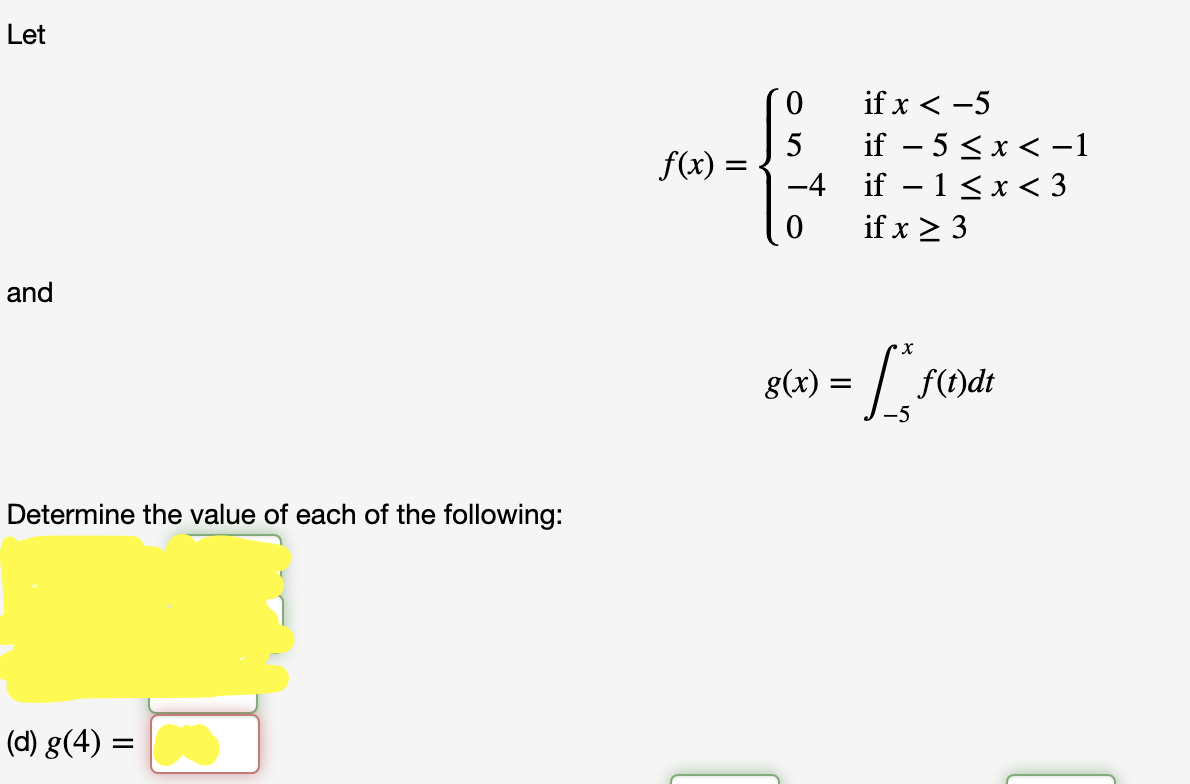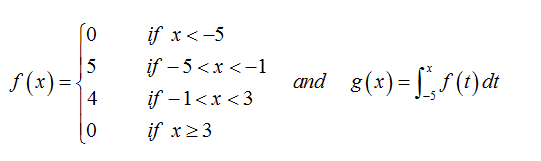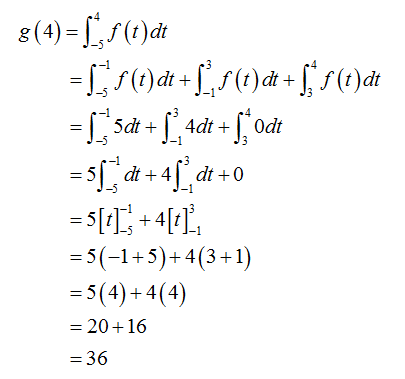# Letif x < -5if – 5 < x < -1– 1 < x < 3if x > 3f(x) =-4andх8(х) —f(t)dt-5Determine the value of each of the following:(d) g(4) =

Question
1 viewshelp_outlineImage TranscriptioncloseLet if x < -5 if – 5 < x < -1 – 1 < x < 3 if x > 3 f(x) = -4 and х 8(х) — f(t)dt -5 Determine the value of each of the following: (d) g(4) = fullscreen
check_circle

Step 1

Given functionStep 2

Now g (4) is given by...

### Want to see the full answer?

See Solution

#### Want to see this answer and more?

Solutions are written by subject experts who are available 24/7. Questions are typically answered within 1 hour.*

See Solution
*Response times may vary by subject and question.
Tagged in